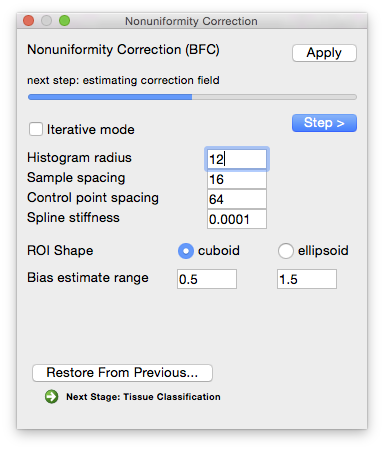Select Page

# bfc: compute pointwise spline estimatesThis stage will compute a set of initial estimates on sub-volume blocks with half-width equal to the histogram radius, in voxels. For anisotropic voxels, this sub-volume is scaled in each direction to correspond to the same physical size based on the smallest dimension of the voxel. For example, a histogram radius of 12 with a voxel size of 1mm x 1mm x 1.5mm would specify a box that is 25 x 25 x 17 voxels (25 = 2 x 12 + 1, 17 = 2 x 8 + 1).

### Parameters

Histogram radius (in voxels—typical range: 8–32)
The half-width of sub-volume used for sampling, which determines how well-localized the estimate is. A larger radius provides more data to estimate the gain variation, but it also includes a larger area and the gain estimate may not be appropriate for all areas of the sub-volume. A smaller radius will provide a more localized estimate, but has fewer data points to fit. In general, a conservative setting is selected for this value. Increasing the size of the radius will produce smoother estimate fields. Decreasing the value will increase the variations seen in the local gain estimates. It is important to note that the spline-fitting procedure may smooth the field even more.
Sample spacing (in voxels)
The size of the sample spacing determines how many point estimates are used throughout the image. For smoother gain fields, this value can be increased to produce a sparser sampling. For rapidly changing fields, this number should be decreased to allow more frequent sampling of the gain field.
ROI shape
Shape of the blocks used for estimating local bias level.